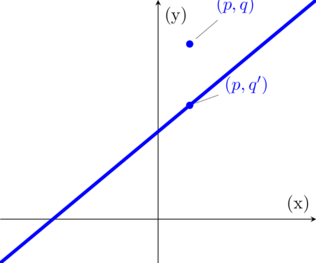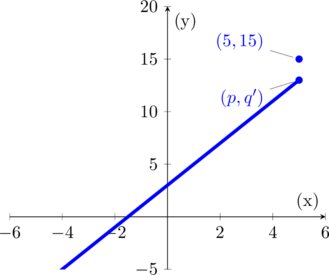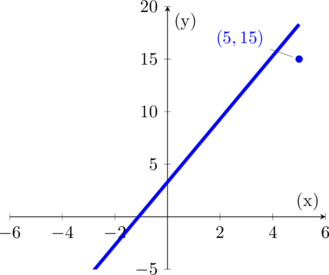# Square Trick for Linear Regression in ML

This is part of my notes taken while studying machine learning. I’m learning as I go along, and may update these with corrections and additional info.

In my Linear Regression for ML notes, we discussed need way to move the line to best fit our data. Two methods for doing so are:

### Square Trick

Similar to the absolute trick, but takes into account the distance the line is from the point. If the line is a small distance away from the point, we want to move it a small amount. If it is a large distance away, move it a larger amount.

y = (w1 – p(q-q’)⍺)x + (w2 + (q-q’)⍺)

q’ = (called q prime) is y position of where the point is located on the line. So q – q’ is the vertical distance between the point and the line.### Square Trick Example

Using our same example from the absolute trick, let’s do it again using square trick. Our line is currently at y = 2x + 3 and we want to move it closer to the point at (5, 15).With a learning rate of 0.1, we are going to use the square trick expression:

y = 2x + 3 starting expression

y = (w1 + p(q-q’)⍺)x + (w2 + (q-q’)⍺) move it to this

y = (2 + (5 x (15-13) x 0.1))x + (3 + ((15-13)x0.1)) add our learning rate and p’

y = 3x + 3.3 and now we have this.

When we plot our new expression, the line is getting closer to our point: